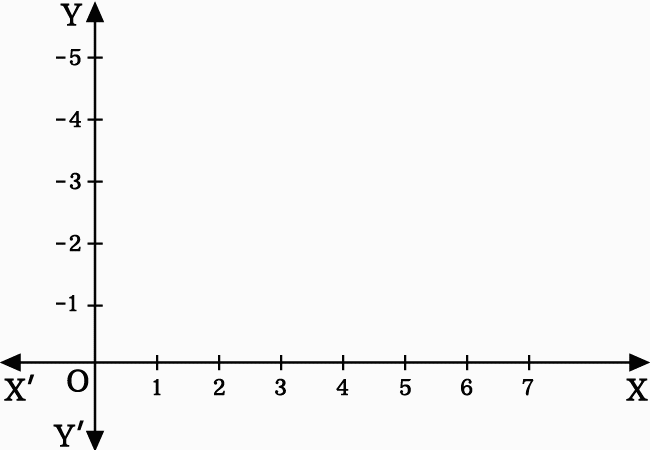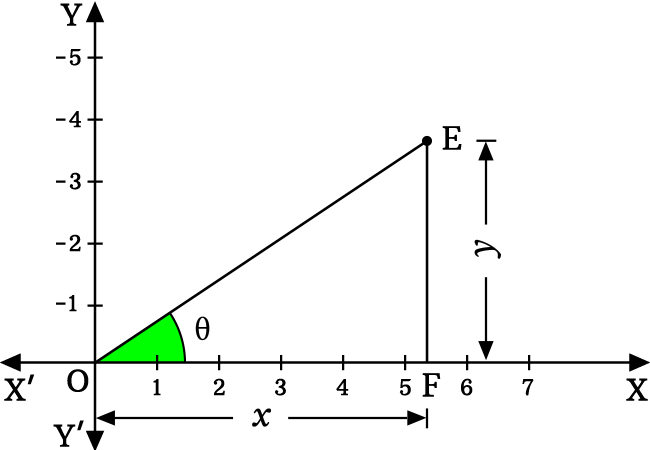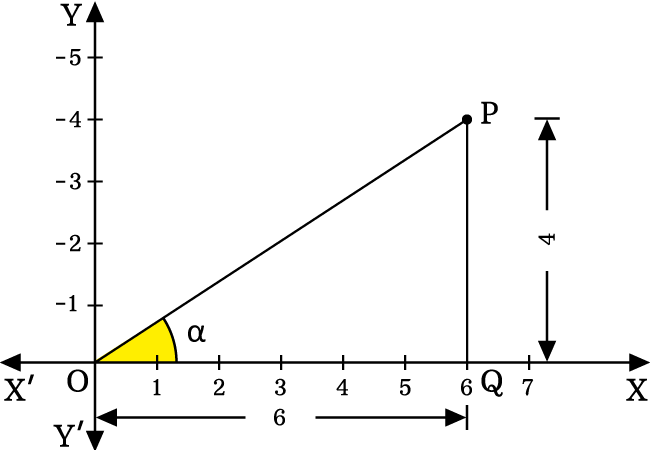# Sign of Tan function in first quadrant

## Sign

$\sin{\theta} \, > \, 0$

The sign of tan function in first quadrant of two-dimensional space is positive.

### Proof

The sign of tan function in first quadrant of two-dimensional Cartesian coordinate system can be proved geometrically. It helps us to understand how the sign of tangent function in first quadrant is positive.1. Let $E$ be a point in first quadrant of two-dimensional Cartesian coordinate system.
2. Connect the point $E$ with the origin of two-dimensional space by a line.
3. Draw a perpendicular line to horizontal axis from point $E$ and it intersects the $x$-axis at point $F$.

Thus, a right triangle is constructed geometrically and let’s take the angle of $\Delta EOF$ as theta ($\theta$). Therefore, the tangent function is written as $\tan{\theta}$ in trigonometry.

Let’s take the length of adjacent side is denoted by $x$ and the length of opposite side is represented by $y$. Now, express the tan function in ratio form of lengths of sides.

$\tan{\theta} \,=\, \dfrac{EF}{OF}$

In this case, $EF = y$ and $OF = x$.$\implies \tan{\theta} \,=\, \dfrac{y}{x}$

In this case, the variable $x$ represents any value on the $x$-axis. Similarly, the variable $y$ represents any value on the $y$-axis. Actually, the $x$-axis and $y$-axis both represent positive values in first quadrant. Therefore, the lengths of opposite and adjacent sides should be positive. Therefore, $x > 0$ and $y > 0$.

$\implies \tan{\theta} \,=\, \dfrac{y}{x}$

In first quadrant, the values of $x$ and $y$ are positive. So, the quotient of them should also be positive. Therefore, the sign of tan function value is also positive.

$\,\,\, \therefore \,\,\,\,\,\, \tan{\theta} \, > \, 0$

### Example

$\Delta POQ$ is a right triangle in first quadrant of two dimensional space. The angle of this triangle is $\alpha$. The tangent function can be expressed in ratio form of lengths of sides.$\tan{\alpha} \,=\, \dfrac{PQ}{OQ}$

In this example, $PQ \,=\, 4$ and $OQ \,=\, 6$. The sign of length of each side is positive. Now, substitute the lengths of sides in the ratio for calculating the tan value.

$\implies \,\,\,$ $\tan{\alpha} \,=\, \dfrac{4}{6}$

$\require{cancel} \implies \,\,\,$ $\tan{\alpha} \,=\, \dfrac{\cancel{4}}{\cancel{6}}$

$\implies \,\,\,$ $\tan{\alpha} \,=\, \dfrac{2}{3}$

The value of tan function $\tan{\alpha}$ is positive and it can be understood that the sign of tan function in first quadrant is positive.

Latest Math Topics
Jun 26, 2023
Jun 23, 2023

Latest Math Problems
Jul 01, 2023
Jun 25, 2023
###### Math Questions

The math problems with solutions to learn how to solve a problem.

Learn solutions

Practice now

###### Math Videos

The math videos tutorials with visual graphics to learn every concept.

Watch now

###### Subscribe us

Get the latest math updates from the Math Doubts by subscribing us.